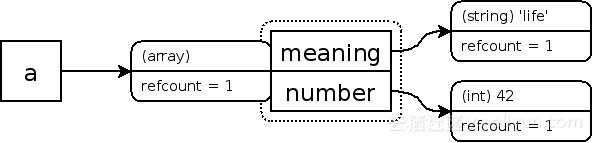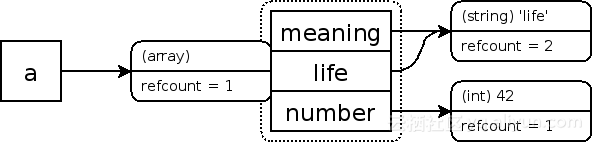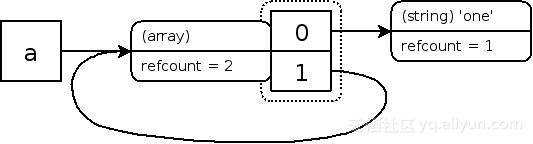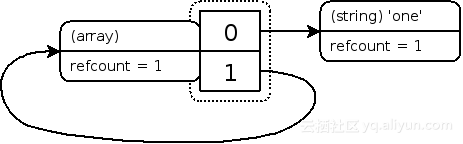#php的垃圾回收机制——引用计数

0
1
01. 云栖社区>
2. 博客>
3. 正文

## php的垃圾回收机制——引用计数

yuliang.lyl 2017-09-30 22:44:50 浏览4478

``````<?php
\$a = "new string";
?>``````

``````<?php
xdebug_debug_zval('a');
?>``````

a: (refcount=1, is_ref=0)='new string'

``````<?php
\$a = "new string";
\$b = \$a;
xdebug_debug_zval( 'a' );
?>``````

a: (refcount=2, is_ref=0)='new string'

``````<?php
\$a = "new string";
\$c = \$b = \$a;
xdebug_debug_zval( 'a' );
unset( \$b, \$c );
xdebug_debug_zval( 'a' );
?>``````

a: (refcount=3, is_ref=0)='new string'
a: (refcount=1, is_ref=0)='new string'

``````<?php
\$a = array( 'meaning' => 'life', 'number' => 42 );
xdebug_debug_zval( 'a' );
?>``````

a: (refcount=1, is_ref=0)=array (
'meaning' => (refcount=1, is_ref=0)='life',
'number' => (refcount=1, is_ref=0)=42
)``````<?php
\$a = array( 'meaning' => 'life', 'number' => 42 );
\$a['life'] = \$a['meaning'];
xdebug_debug_zval( 'a' );
?>``````

a: (refcount=1, is_ref=0)=array (
'meaning' => (refcount=2, is_ref=0)='life',
'number' => (refcount=1, is_ref=0)=42,
'life' => (refcount=2, is_ref=0)='life'
)``````<?php
\$a = array( 'meaning' => 'life', 'number' => 42 );
\$a['life'] = \$a['meaning'];
unset( \$a['meaning'], \$a['number'] );
xdebug_debug_zval( 'a' );
?>``````

a: (refcount=1, is_ref=0)=array (
'life' => (refcount=1, is_ref=0)='life'
)

``````<?php
\$a = array( 'one' );
\$a[] =& \$a;
xdebug_debug_zval( 'a' );
?>``````

a: (refcount=2, is_ref=1)=array (
0 => (refcount=1, is_ref=0)='one',
1 => (refcount=2, is_ref=1)=...
)(refcount=1, is_ref=1)=array (
0 => (refcount=1, is_ref=0)='one',
1 => (refcount=1, is_ref=1)=...
)Zvals after removal of array with a circular reference demonstrating the memory leak

yuliang.lyl
+ 关注